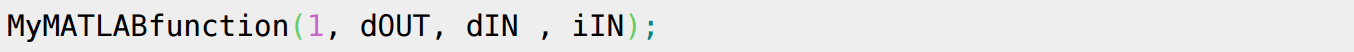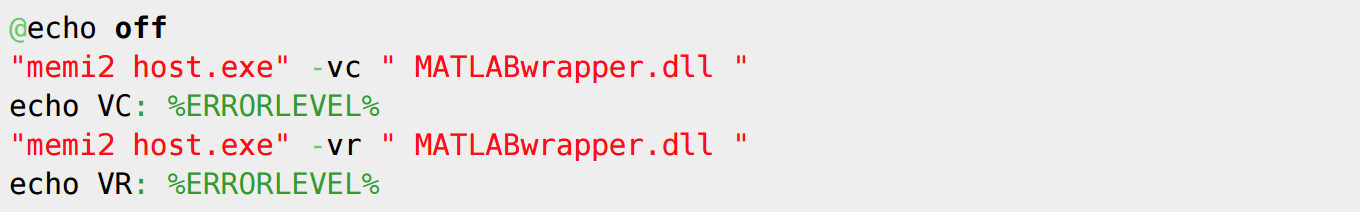'. PHP_EOL; } elseif ( strpos( \$page_path, "deutsch") !== false) { echo ''. PHP_EOL; } elseif ( strpos( \$page_path, "francais") !== false) { echo ''. PHP_EOL; } elseif ( strpos( \$page_path, "italiano") !== false) { echo ''. PHP_EOL; } ?>

# Integrating MATLAB Models with Mimic Using MEMI

by | Dec 4, 2009 | Simulation# Mart Berutti

## VP, Digital Transformation Sales & Marketing

The purpose of this technical note is to demonstrate how to use a model developed in MathWorks MATLAB with Mimic Simulation Software using the Mimic External Modeling Interface (MEMI).

Using the MATLAB Compiler, it is possible to create a shared library from MATLAB code written as an m-file, known as a Dynamic Link Library, which can be run outside of MATLAB. The current MEMI version allows DLLs to run in Mimic. Since variables in MATLAB are treated as matrices, it would be impossible to directly compile MATLAB code into something that is useable by MEMI but it is possible to create a DLL wrapper that executes the MATLAB shared library.

BUILDING THE MATLAB SHARED LIBRARY

A simple MATLAB function was created to demonstrate compiling MATLAB functions into a shared library.This function will output a single X position based on the inlet variables. The arguments are separated as Adoubles and Bints because matrices in MATLAB can only handle one data type.

The MATLAB function is compiled by typing in the MATLAB command window:Executing the command will generate several important files:

• lib & myMATLABcompiled.h are necessary for compiling your C++ wrapper DLL.
• dll will be executed by your C++ wrapper DLL.
• txt provides further details on using the MATLAB DLL such as running it on another machine.

BUILDING THE WRAPPER SHARED LIBRARY

Since MEMI 2 can not directly run the MATLAB DLL, an addition shared library will need to be created to coordinate between MEMI2 and the MATLAB DLL.

The C++ code created for the MATLAB DLL wrapper has a few essential parts. In the InitializeMEMI function, the MATLAB runtime application and the MATLAB library need to be initialized.During the ExecuteMEMI function, the MATLAB function arguments must be declared as mwArray variables. Searching the internet for mwArray will provide more information for handling the special MATLAB data types.After data is loaded into the mwArray varibles, the MATLAB function can finally be executed.In the CleanUpMEMI function, close the library and applicationTo compile it, it must be placed in the MATLAB current folder. The command to compile it isCompiling it will generate the file MATLABwrapper.dll

CONFIGURING MIMIC

Begin by copying both .dll files (myMATLABcompiled.dll & MATLABwrapper.dll) into the mimic folder (C:\Program Files\MYNAH Technologies\Mimic). This is necessary for the myMATLABcompiledInitialize() function to execute.

After they are in place, open Mimic Explorer as administrator and navigate the External Models section in your chosen node. Create a new Definition, and under it, create a new model. Right click on the definition and select properties. In the section labeled “DLL FILE PATH:” select the ellipsis and navigate to your wrapper DLL. A popup should appear that prompts the user to replace the Existing Variables.

If MEMI2 provides you with a pop-up error, you can try debugging the issue by running the following commands as a batch file:USING MIMIC THE MATLAB DLL ON A SEPARATE MACHINE

To run the MATLAB DLL on a separate machine, you will need to install the MATLAB runtime. Install the MATLAB runtime by following the directions in readme.txt, which is found by compiling the MATLAB code.

64-BIT/32-BIT LIMITATION

At the time of writing this, MATLAB is only sold as a 64-bit version, but Mimic is available in 32-bit and 64-bit versions. MATLAB normally only compiles as 64-bit code and therefore can only create DLL that works with 64-bit Mimic.

SOFTWARE REQUIREMENTS

The MEMI functionality used to integrate Matlab models is available in Mimic v3.6.2 or later.

According to the MathWorks website, the the necessary components for Matlab would be:

• MATLAB Compiler
• MATLAB Compiler SDK